Displaying Mathematical Notations in eClass Article Last Updated June 2021 There are 2 main methods of displaying mathematical notations in eClass, depending on users familiarity with math markup languages: the more robust method uses the MathJAX filter to display standard TeX syntax (or MathML); users unfamiliar with TeX can use an editor tool to add preformatted equations and syntax. Displaying TeX Using the MathJAX filter You can enable the filter at your course level (if you want mathematical notations throughout the course) by clicking on the gear icon in the top right of your course header, then on Filters. Scroll down to find ‘MathJax’ and turn the filter on.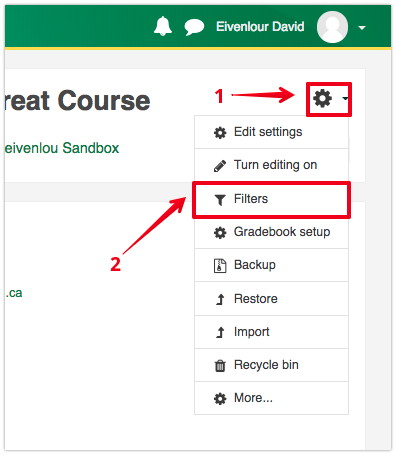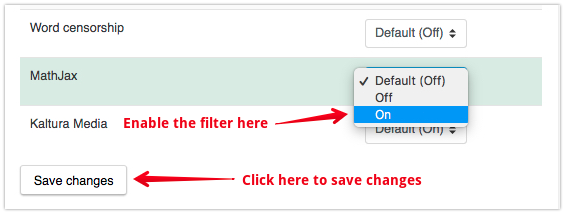You can also enable the filter at the context of a specific activity (if you only plan to use the notation in that specific place). Go to the activity page, click on the gear icon, then on Filters. As the conversion of the TeX syntax into mathematical notation requires calls to other files on eClass, it is always recommended to turn the filter on only at the contexts where it is required.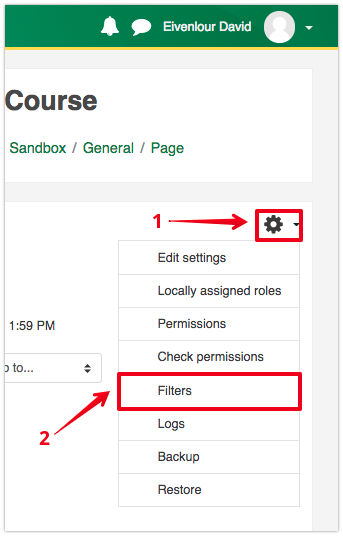In the html editor for the Page content of the page resource, enter whatever text desired. Wherever you require mathematical notation, begin and end the TeX syntax with the following: For expressions to be listed inline with text, use $$...$$ For expressions to be displayed independently, use $$...$$ or $...$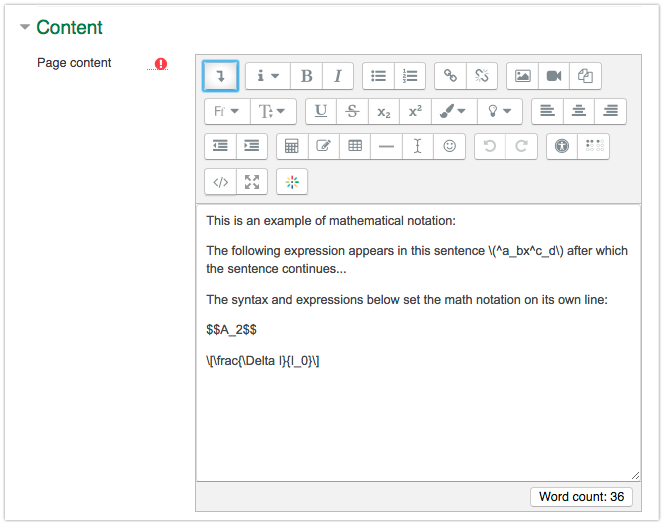In the example above, the full syntax of the math notations is: $$^a_bx^c_d$$ $$A_2$$ $\frac{\Delta l}{l_0}$ In the page on eClass, this syntax gets displayed as the following: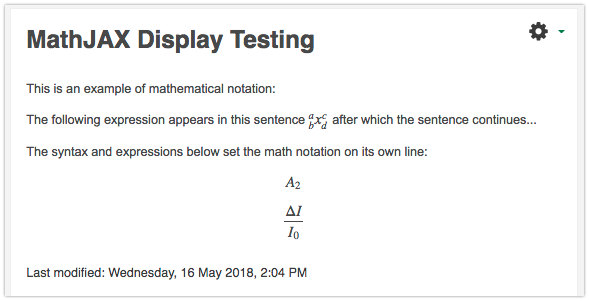Basic Editor Equation Option If you are unfamiliar with TeX notation or would like to preview an equation, eClass has a built in equation editor: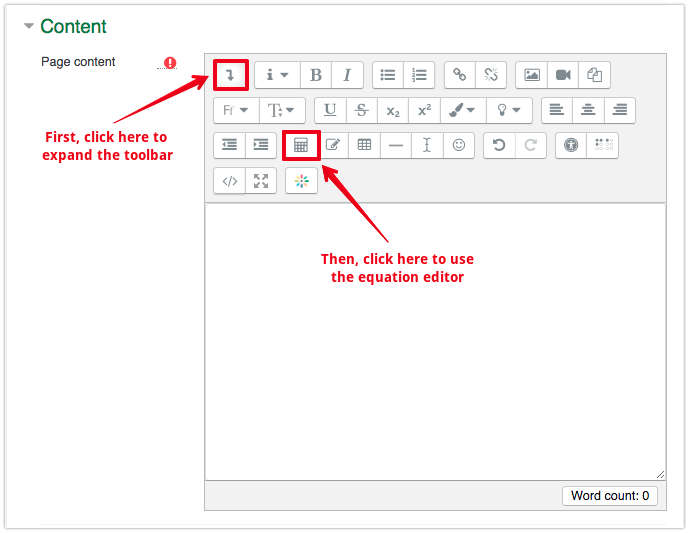In the Equation preview, the ⇓ character indicates the approximate location of your cursor in the equation. If instead of seeing the ⇓ icon, you see the word “Downarrow”, your cursor is likely in an invalid position.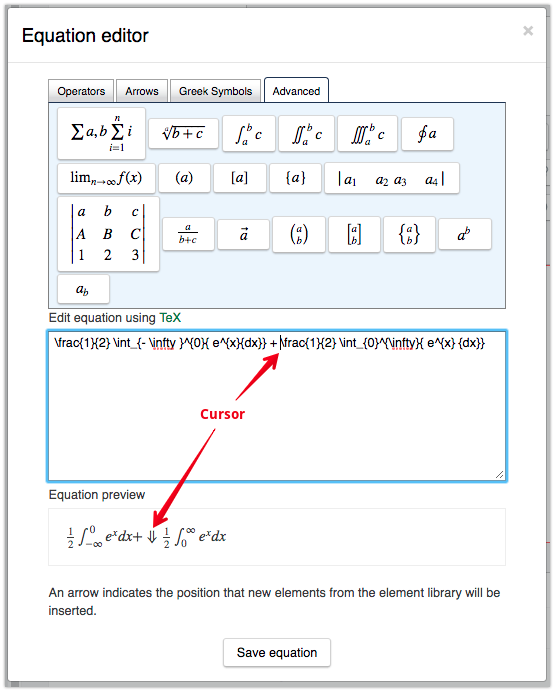At the top of the Equation editor, there are Operations, Arrows, Greek Symbols, and Advanced formulas pre-built that can be added to an equation. Numbers, letters, and operators can be added manually under Edit equation using TeX.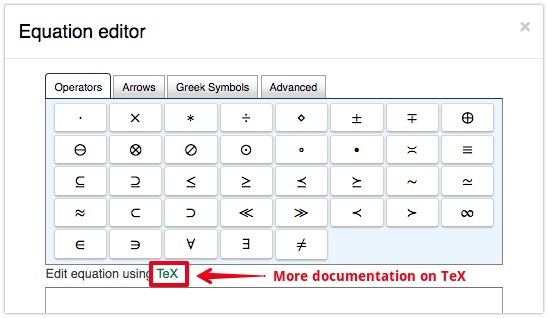To leverage the full potential of mathematical notation in eClass, custom TeX formulas can be inputted and previewed in the equation editor. To view an existing equation in the equation editor, place your cursor anywhere within the TeX expression and select the Equation editor button. For more information on the TeX mathematical notations available in MathJAX, see the following page: https://docs.moodle.org/31/en/Using_TeX_Notation(6 vote(s))HelpfulNot helpful